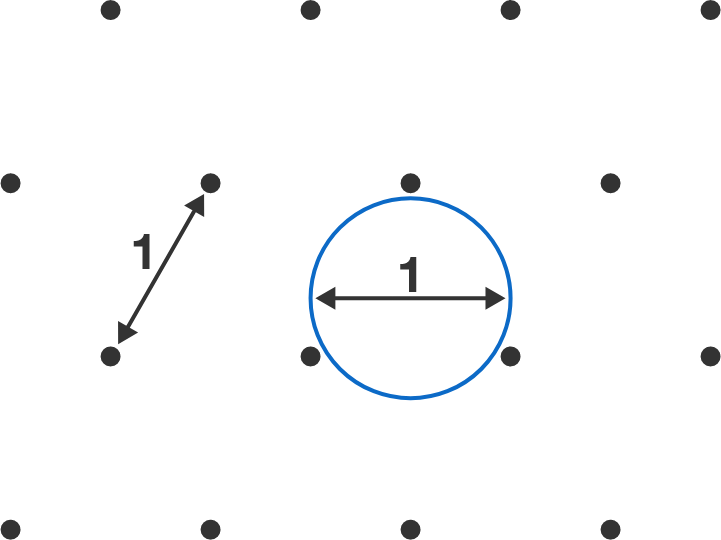# Problems of the Week

Contribute a problem

# 2018-04-23 Intermediate

If $\frac {a + b}c = \frac 65$ and $\frac {b + c}a = \frac 92,$ then what is the value of $\frac {a + c}b?$

Five monkeys in a circus are sitting in the following order:The ringmaster wants to reverse their order, but only by swapping the positions of two monkeys at a time.

This is one way, where two swaps take place:

 1 2 Swap A and E: E B C D A Swap B and D: E D C B A 

Another possible way takes exactly four swaps:

 1 2 3 4 Swap A and E: E B C D A Swap C and D: E B D C A Swap B and D: E D B C A Swap B and C: E D C B A 

Is it possible to do this in exactly three swaps?

The blue figure is made of five quarter-circle arcs (each with integer radius) and a segment of length 4, which is the base of a $4\times 4$ square grid.

What is the area of the blue figure?An infinite triangular lattice consists of points spaced 1 unit apart.

What is the probability (as a percentage) that a circle of unit diameter placed randomly in the lattice encloses no lattice points, as shown?$\frac 1{1},\ \frac {2}{1},\ \frac {1}{2},\ \frac {3}{1},\ \frac {2}{2},\ \frac {1}{3},\ \frac {4}{1},\ \frac {3}{2},\ \frac {2}{3},\ \frac {1}{4},\ \ldots$

If $\frac {1887}{1920}$ is the $m^\text{th}$ term and $\frac {1789}{2018}$ is the $n^\text{th}$ term of the sequence above, find $|m-n|.$

Note: In this sequence, the fractions do not reduce. For example, $\frac{2}{2}$ is distinct from $\frac{1}{1}.$

×

Problem Loading...

Note Loading...

Set Loading...